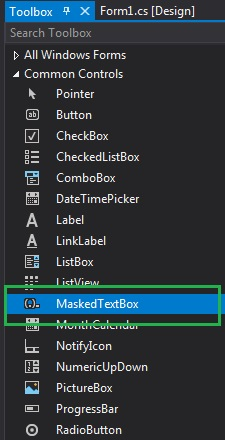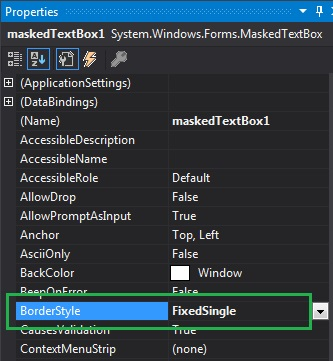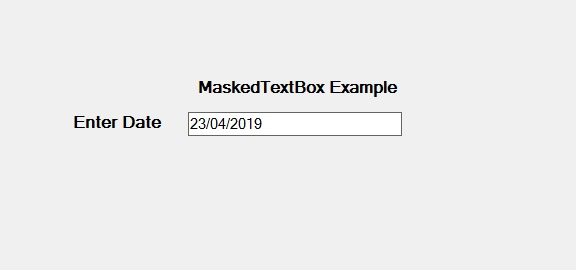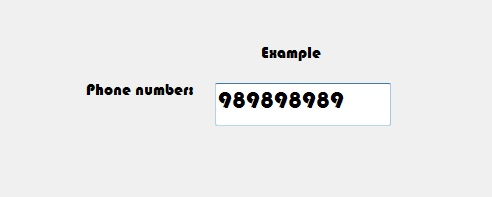# How to style the Border of the MaskedTextBox in C#?

In C#, MaskedTextBox control gives a validation procedure for the user input on the form like date, phone numbers, etc. Or in other words, it is used to provide a mask which differentiates between proper and improper user input. In MaskedTextBox control, you can style the border of the MaskedTextBox using BorderStyle Property. This property has three different values that are defined under BorderStyle enum and the values are:

• None value for no border.
• Fixed3D value for 3-D border.
• FixedSingle value for single line border.

The default value of this property is Fixed3D. You can set this property in two different ways:

1. Design-Time: It is the easiest way to set the border style of the MaskedTextBox as shown in the following steps:

• Step 1: Create a windows form as shown in the below image:
Visual Studio -> File -> New -> Project -> WindowsFormApp• Step 2: Next, drag and drop the MaskedTextBox control from the toolbox on the form as shown in the below screenshot:• Step 3: After drag and drop you will go to the properties of the MaskedTextBox and set the border style of the MaskedTextBox as shown in the below image:Output:2. Run-Time: It is a little bit trickier than the above method. In this method, you can set the border style of the MaskedTextBox control programmatically with the help of given syntax:

`public System.Windows.Forms.BorderStyle BorderStyle { get; set; }`

Here, BorderStyle represents the border type of the MaskedTextBox control. It will throw an InvalidEnumArgumentException if the value of this property does not belong to BorderStyle property. The following steps show how to set the border style of the MaskedTextBox dynamically:

```// Creating a MaskedTextBox
```
• Step 2: After creating MaskedTextBox, set the BorderStyle property of the MaskedTextBox provided by the MaskedTextBox class.
```// Setting the BorderStyle
m.BorderStyle = BorderStyle.Fixed3D;
```
• Step 3: And last add this MaskedTextBox control to the form using the following statement:
```// Adding MaskedTextBox control on the form
```

Example:

 `using` `System; ` `using` `System.Collections.Generic; ` `using` `System.ComponentModel; ` `using` `System.Data; ` `using` `System.Drawing; ` `using` `System.Linq; ` `using` `System.Text; ` `using` `System.Threading.Tasks; ` `using` `System.Windows.Forms; ` ` `  `namespace` `WindowsFormsApp36 { ` ` `  `public` `partial` `class` `Form1 : Form { ` ` `  `    ``public` `Form1() ` `    ``{ ` `        ``InitializeComponent(); ` `    ``} ` ` `  `    ``private` `void` `Form1_Load(``object` `sender, EventArgs e) ` `    ``{ ` `        ``// Creating and setting the  ` `        ``// properties of the Label ` `        ``Label l1 = ``new` `Label(); ` `        ``l1.Location = ``new` `Point(413, 98); ` `        ``l1.Size = ``new` `Size(176, 20); ` `        ``l1.Text = ``" Example"``; ` `        ``l1.Font = ``new` `Font(``"Bauhaus 93"``, 12); ` ` `  `        ``// Adding label on the form ` `        ``this``.Controls.Add(l1); ` ` `  `        ``// Creating and setting the ` `        ``// properties of Label ` `        ``Label l2 = ``new` `Label(); ` `        ``l2.Location = ``new` `Point(242, 135); ` `        ``l2.Size = ``new` `Size(126, 20); ` `        ``l2.Text = ``"Phone number:"``; ` `        ``l2.Font = ``new` `Font(``"Bauhaus 93"``, 12); ` ` `  `        ``// Adding label on the form ` `        ``this``.Controls.Add(l2); ` ` `  `        ``// Creating and setting the  ` `        ``// properties of the MaskedTextBox ` `        ``MaskedTextBox m = ``new` `MaskedTextBox(); ` `        ``m.Location = ``new` `Point(374, 137); ` `        ``m.Mask = ``"000000000"``; ` `        ``m.Size = ``new` `Size(176, 20); ` `        ``m.Name = ``"MyBox"``; ` `        ``m.BorderStyle = BorderStyle.Fixed3D; ` `        ``m.Font = ``new` `Font(``"Bauhaus 93"``, 18); ` ` `  `        ``// Adding MaskedTextBox  ` `        ``// control on the form ` `        ``this``.Controls.Add(m); ` `    ``} ` `} ` `} `

Output:My Personal Notes arrow_drop_upCheck out this Author's contributed articles.

If you like GeeksforGeeks and would like to contribute, you can also write an article using contribute.geeksforgeeks.org or mail your article to contribute@geeksforgeeks.org. See your article appearing on the GeeksforGeeks main page and help other Geeks.

Please Improve this article if you find anything incorrect by clicking on the "Improve Article" button below.

Article Tags :

Be the First to upvote.

Please write to us at contribute@geeksforgeeks.org to report any issue with the above content.